#DAY 3
4
Software Development

## Day3 - 天啊我只有「螢幕十行」，python簡力救救我啊!

(學校修課寫約數百~一千行的程式期末專案也可以算)

「螢幕十行」導致必須上下捲動螢幕才可以看到完整的程式碼，

python能以很簡短的程式碼表達完整的功能，

# 到底python語法上精簡了什麼?

## 從HelloWorld說起

HelloWorld通常是學任何一門程式語言的第一堂課- 「在螢幕上印出一行字- HelloWorld」
，象徵著你踏上學習程式的旅程了。

``````#include <stdio.h>
int main()
{
printf("Hello world!\n");
return 0;
}
``````

``````public class HelloWorld {
public static void main(String[] args) {
System.out.println("Hello! World!");
}
}
``````

`public`, `class`, `static`, `void`

python的HelloWorld 一行即可:

``````print("HelloWorld")
``````

## 特性二: 一句話的結束不必加分號

，表示一個句字的結束。

``````print("HelloWorld")
``````

``````print("HelloWorld");
``````

## 特性三: 以縮排區分程式碼內外區域

``````#include <stdio.h>

int main()
{
int x=3;
int y=1;
if(x==3)
{
printf("x=3\n");
y=5;
}
printf("y=%d\n",y);
}
``````

``````# python程式
x, y =3, 1
if x==3:
print("x=3")
y=5
print("y=",y)
``````

## 特性四: 變數不必先宣告型態

``````s=2
print(type(s))
s= "python"
print(type(s))
``````

`<class 'int'>`
`<class 'str'>`

``````myList=[True, "abc", 50, 6.3, [1,2,5]]
``````

## 特性五: 可一次修改多個變數的值

``````//C程式
int x,y,z;
x=1;
y=2;
z=3;
``````

``````# python程式
x,y,z=1,2,3
``````

### 範例3-1: 交換兩個變數a,b的值

``````int a=3, b=5;
int temp=a;
a=b;
b=temp;
``````

``````a=b;
b=a;
``````

``````a,b=b,a
``````

## 特性六: 所有變數名稱其實就像c的指標

### 範例3-2: 誰偷吃了我的蛋糕?

``````fridge = ['蛋糕', '蘋果', '香蕉']
b = fridge
b.remove('蛋糕')
print("列表b的內容為:", b)
print("列表fridge的內容為:", fridge)
``````

['蛋糕', '蘋果', '香蕉']是裝有三個字串的列表，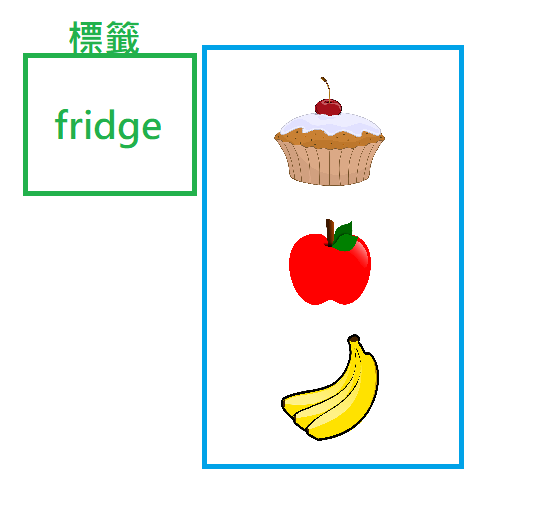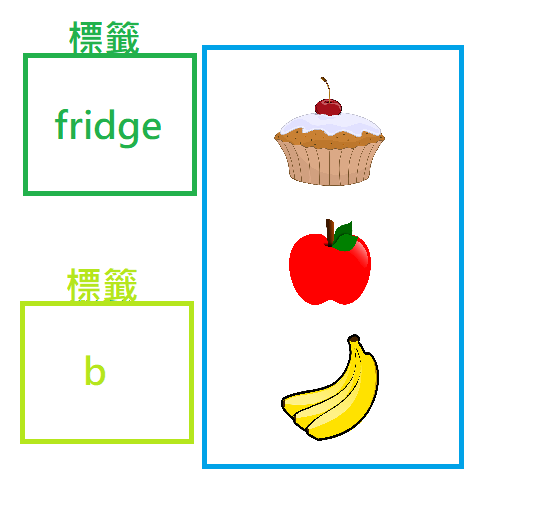(廢話~ 是同一個冰箱嘛~ 如圖示)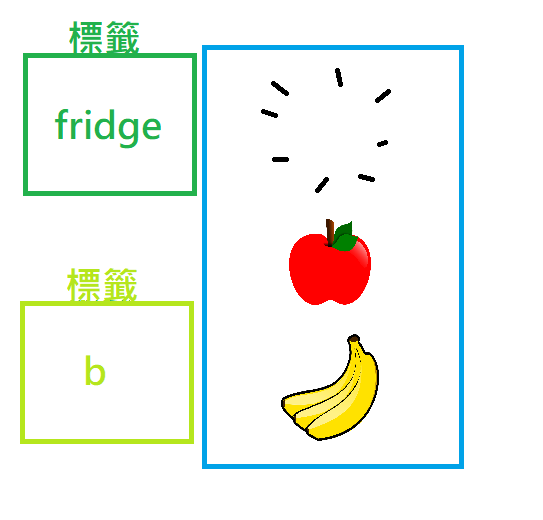### 範例3-3: 貼到別的冰箱

``````fridge = ['蛋糕', '蘋果', '香蕉']
b = fridge
b = ['蘋果', '香蕉']
print("列表b的內容為:", b)
print("列表fridge的內容為:", fridge)
``````

`列表b的內容為: ['蘋果', '香蕉']`
`列表fridge的內容為: ['蛋糕', '蘋果', '香蕉']`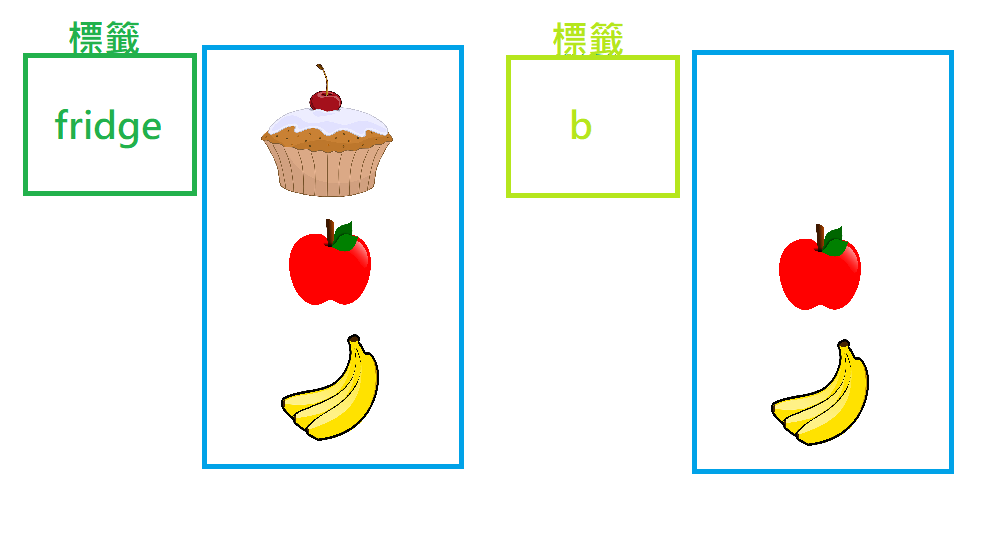### 那那…究竟如何真的複製一個冰箱呢?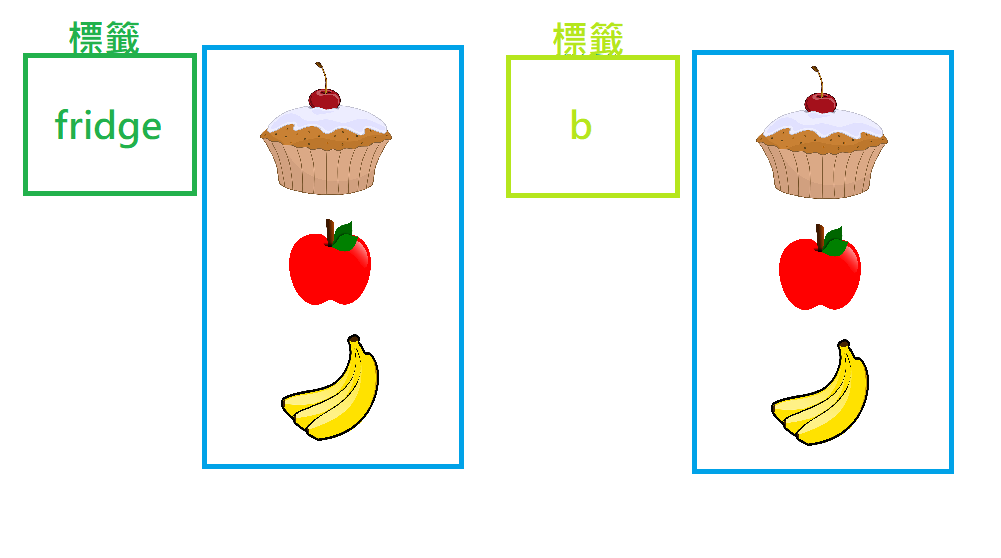`b = fridge`改成
`b = fridge[:]`就好，

``````fridge = ['蛋糕', '蘋果', '香蕉']
b = fridge[:]
b.remove('蛋糕')
print("列表b的內容為:", b)
print("列表fridge的內容為:", fridge)
``````

`列表b的內容為: ['蘋果', '香蕉']`
`列表fridge的內容為: ['蛋糕', '蘋果', '香蕉']`

# 伏筆?

• 你剛剛寫的`b = fridge[:]`為什麼可以這樣寫?
• 你在Day1秀的那個三行程式變一行的那個魔法
``````L=[i for i in range(1,101)]
``````

• 一直在學python語法，好像還沒看到python特別出色的地方啊?